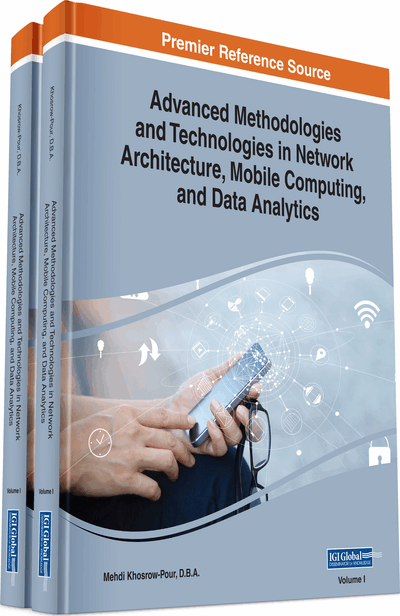# An Essay on Denotational Mathematics

Giuseppe Iurato (University of Palermo, Italy)
DOI: 10.4018/978-1-5225-7598-6.ch120
Available
\$37.50
No Current Special Offers

## Abstract

Denotational mathematics is a new rigorous discipline of theoretical computer science that springs out from the attempt to provide a suitable mathematical framework in which laid out new algebraic structures formalizing certain formal patterns coming from computational and natural intelligence, software science, cognitive informatics, neuronal networks, and artificial intelligence. In this chapter, a very brief but rigorous exposition of the main formal structures of denotational mathematics is outlined within naive set theory.
Chapter Preview
Top

## Introduction

Denotational Mathematics (in short, DM) is a new discipline which serves as a formal language to lay out and solve the various problems posed, amongst others, by cognitive informatics, software science and computational intelligence. It has been worked out in the last decades by Yingxu Wang (2008a-e, 2009a-c, 2010a-b, 2011, 2012a-b)1, to whose many works and papers we refer for a wider and more complete information science contextualization of DM and its applications.

In this contribution, we wish to briefly outline, but in a rigorous manner, the main formal structures considered by DM, within the framework of classical algebra of relations. Our exposition is extremely technical and synthetic to allow a faster and clearer overall view of the pivotal mathematical structures of DM, which are concept algebra, real-time process algebra, system algebra, visual semantic algebra, granular algebra, and inference algebra.

In relation to its possible applications to the general context of information sciences, numerical mathematics, as regard continuous context, mainly deals with rational (), real () and complex () number systems, while, as regard discrete context, it deals with natural (), integral () and binary () numerical systems. DM may be considered either from the classical algebra viewpoint (as done here) or from the model theory one2. In any case, it mainly deals with hypercomplex () denotational algebraic systems, which will be defined in the next section 4.

Top

## Background

Generally speaking, methods of mathematics may be roughly classified into analytical, numerical, and denotational approaches. The analytical approach deals with continuous mathematical objects defined in the real number field (); the numerical approach deals with discrete mathematical entities defined inand in binary systems () with iteratively and recursively approximation functions.

However, the domains of recent problems in the emerging disciplines of artificial intelligence, cognitive informatics, brain science, cognitive computing, knowledge science, and computational intelligence require rather new complex hyperstructures (), beyond that of pure numbers in () and (), which just identify the denotational approach.

The requirement for reducing complicated formal problems and solutions to the low-level objects in conventional computing theories and the underpinning of analytical or numerical mathematical means, has greatly constrained the inference and computing ability of both human and intelligent systems.

## Complete Chapter List

Search this Book:
Reset